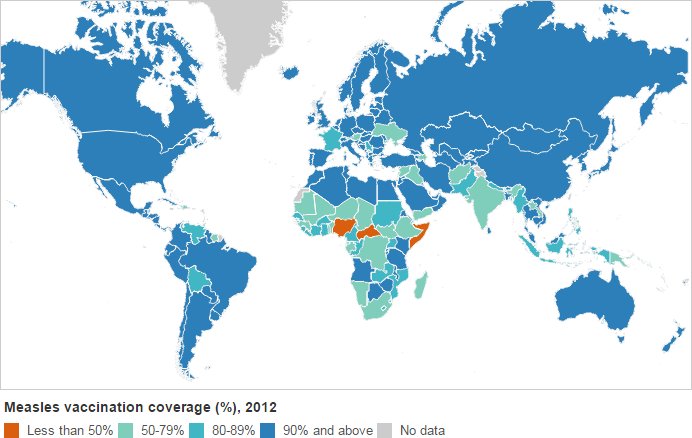Vaccinations are not without risk, some say. Why should the evil government compel me to expose my child to a known risk, they ask. Why is it my problem if someone else’s child is not vaccinated, they argue.

Well… it boils down to simple math, really. Suppose that once infected, a person remains infectious for a period of time denoted by $$\delta t$$. The virulence of the disease is measured by the number of people that a single patient can infect during this period of time. It is called the basic reproduction number, denoted by $$R_0$$. If this number is greater than one, we have the potential for an epidemic.

So then, after some time $$t$$ has elapsed, the number $$N(t)$$ of people who are infectious at that moment $$N(t)=N_0R_0^{t/\delta t}$$, if $$N_0$$ was the number of infected people at $$t=0$$. At least this will be the correct number during the early stages of the epidemic; later, infection rates slow down as a growing number of people will have already caught the disease and the survivors will have developed immunity to it.

But we are interested in the early stages indeed, because the idea is to prevent an epidemic in the first place. So this simple model is adequate.

Now what happens when you vaccinate people? Even if everyone gets vaccinated, vaccines are not 100% effective. If the efficiency of a vaccine is given by $$\epsilon$$ (a number between 0 and 1, with 1 meaning 100% efficiency), the aforementioned formula is modified: $$N(t)=[(1-\epsilon)R_0]^{t/\delta t}$$. If $$(1-\epsilon)R_0<1$$, we win: an epidemic is avoided.

But what happens when not everyone gets vaccinated? Some people obviously cannot be: very young babies, people with compromised immune systems, etc. Let’s say the vaccination rate is given by $$\rho$$. Once again, the formula for $$N(t)$$ needs to be revised: $$N(t)=[(1-\epsilon\rho)R_0]^{t/\delta t}$$.

And this is where the problem lies: if $$(1-\epsilon\rho)R_0>1$$, the potential for an epidemic exists.Take the case of measles, for which $$R_0=12…18$$. Even if we take the lower limit of the given range, $$R_0=12$$, it is one of the most virulent contagious diseases out there. The measles vaccine is supposedly 95% effective: $$\epsilon=0.95$$. So then, $$(1-\epsilon)R_0=0.6$$, and we are good: in a fully vaccinated population, measles would disappear in short order. This is indeed what happened when measles vaccinations became common in much of the world starting in the 1970s.

But now, let us think about $$\rho$$. The math is easy: If $$\rho<0.965$$ (that is, if more than 3.5% of the population are unvaccinated), $$(1-\epsilon\rho)R_0>1$$. Herd immunity is lost: the disease spreads.

And lest we forget, measles is a very deadly disease. Parents who play Russian roulette with their children on the basis of unsubstantiated fears concerning the vaccine’s effectiveness and side effects forget that often the only reason their child survives the infection is because they have access to first-world health care… the same health care that would have prevented the illness in the first place, if not for the parents’ arrogant stupidity.

These parents should be reminded that in poorer parts of the world, their counterparts often risk their lives to get their children vaccinated. Like parents in Somalia who, defying a ban on polio vaccination by al-Shabaab, smuggle their children to government-controlled areas to get the life-saving vaccine. Obviously these Somali parents are a lot smarter, a lot wiser than first-world anti-vaxxers, be it new age parents who prefer “happy thoughts” (or whatever) over medically approved methods, or nutty right-wingers who distrust the government on everything.

In short, if your political or religious views, or your scientific illiteracy compel you to be as stupid as a doorknob, please find a way to express your stupidity without endangering the health and lives of others.